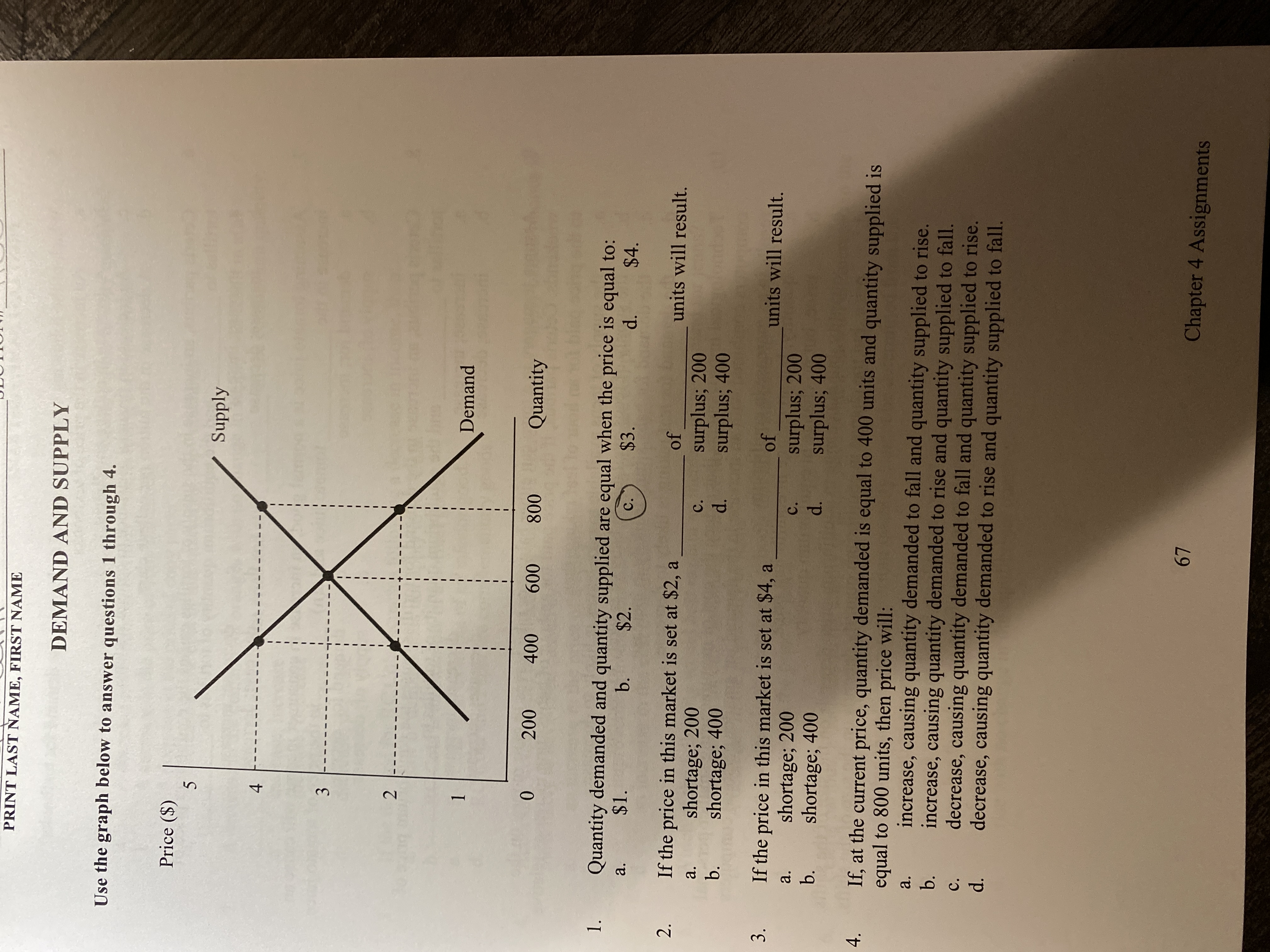# PRINT LAST NAME, FIRST NAMEDEMAND AND SUPPLYUse the graph below to answer questions 1 through 4.Price (\$)5Supply1Demand200400600800Quantity1.Quantity demanded and quantity supplied are equal when the price is equal to:\$1.b.a.\$2.\$3.C.d.\$4.2.If the price in this market is set at \$2, ashortage; 200shortage; 400ofunits will result.a.surplus; 200surplus; 400c.b.d.If the price in this market is set at \$4, ashortage; 200shortage; 4003.ofunits will result.a.surplus; 200surplus; 400C.b.d.If, at the current price, quantity demanded is equal to 400 units and quantity supplied isequal to 800 units, then price will:increase, causing quantity demanded to fall and quantity supplied to rise.increase, causing quantity demanded to rise and quantity supplied to fall.decrease, causing quantity demanded to fall and quantity supplied to rise.decrease, causing quantity demanded to rise and quantity supplied to fall.4.a.b.C.d.67Chapter 4 Assignments4+3.2.

Question
1 views

Question 4help_outlineImage TranscriptionclosePRINT LAST NAME, FIRST NAME DEMAND AND SUPPLY Use the graph below to answer questions 1 through 4. Price (\$) 5 Supply 1 Demand 200 400 600 800 Quantity 1. Quantity demanded and quantity supplied are equal when the price is equal to: \$1. b. a. \$2. \$3. C. d. \$4. 2. If the price in this market is set at \$2, a shortage; 200 shortage; 400 of units will result. a. surplus; 200 surplus; 400 c. b. d. If the price in this market is set at \$4, a shortage; 200 shortage; 400 3. of units will result. a. surplus; 200 surplus; 400 C. b. d. If, at the current price, quantity demanded is equal to 400 units and quantity supplied is equal to 800 units, then price will: increase, causing quantity demanded to fall and quantity supplied to rise. increase, causing quantity demanded to rise and quantity supplied to fall. decrease, causing quantity demanded to fall and quantity supplied to rise. decrease, causing quantity demanded to rise and quantity supplied to fall. 4. a. b. C. d. 67 Chapter 4 Assignments 4+ 3. 2. fullscreen
check_circle

Step 1

It is given that at current price, the quantity demanded is equal to 400 units and the quantity supplied is equal to 800 units. Since the quantity supplied is mroe than the quantity demanded, there is surplus in the economy.

Step 2

So, the price will fall in this case which will induce the consumers to demand more and producers to produce less. This will continue until the quantity demanded is...

### Want to see the full answer?

See Solution

#### Want to see this answer and more?

Solutions are written by subject experts who are available 24/7. Questions are typically answered within 1 hour.*

See Solution
*Response times may vary by subject and question.
Tagged in